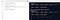# What are Linked List and its basic functions in javascript?

A linked list is a type of Data Structures. Now the question arises what is a data structure and why it is important? So let’s begin with

Data Structures is a collection of data, the way this data is organized in computers so that we can use it effectively, the way we retrieve the data for further processing/manipulation. I hope you are now aware of what is data structures.

Importance: DS is used to manage large amounts of data efficiently in digital space such as databases etc.

Now back to the topic which we are here to discuss mainly;

So, what’s the first thing that came to your mind after seeing the word “linked list”?

A list that contains some data that is linked together through some logic behind it. Linked list elements can be stored anywhere in the memory or randomly stored. Linked lists are used to create trees and graphs.

A single linked list element is known as a node which consists of two parts; data value and a pointer addressing the next node.

In a single linked list, the address of the first node is always stored in a pointer known as “head”(sometimes called front). And the last node of the list points to null.

Now, let's do some basic operations of a Single linked list;

Step 1: create a class representing a linked list. Here methods inside the class are the operations we perform with a linked list. In the constructor, as for now, the list is empty so the tail will be equal to the head node.

Step 2: Insertion method

Create a temporary node tempNode for storing the new value that will be passed to the function for the time being. Its pointer points to null because the new value is always inserted as the last node of the linked list. Now using tail.next, update the tail node and assign the tempNode value to the tail.

Now, check if our code is working or not.

Step 3: Deletion:

Here the first one is remove(value) function, where exsit 3 cases; delete value from start(head), delete node from the end(tail) and delete node anywhere from the linked list between head and tail (with the case if we didn’t find node in the list). Andsecond function is removeTail(), that doesn’t take any arguments and delete the last node.

# i) Deletion from the start (head):

When user passes the value to remove(value) function, check if this value equals to head.value then delete it and now head points towards its next value. So here is the code

# ii) Deletion at the end (tail):

There may exsit 2 cases; Either user directly use removeTail() function if he/she wants to delete last node or if enters the value that stores in last node but he/she didn’t know that.

removeTail():

What we want is to delete the last node (tail) and the node before the last node will now point to null and assigned as the tail. So, what's the logic?

In order to do that, first, create a temporary node currentNode that also points to the head and will traverse the linked list to find the tail using the while loop. When currentNode.next will be tail, the loop will be a break and now we have currentNode pointing second the last node. Assign currentNode to null and currentNode values to the tail. This way, the last node of the list will be deleted.

Let’s Run this code;as you can see the last node containing value 7 is now deleted from the end of the list.

remove(value):

If we the passing value is equals to tail.value, then the logic is same that we use for removeTail() function. So, here is the code:

# iii) deletion within the list:

Now what we want is that if the user input some data value for deletion anywhere from the list. Here are the steps to follow for the deletion that i used my own.

1. search the value in the list if exist by transversing the list
2. if we find the node that equals to value, then swap this node value with next node value until tail, then delete the last node.

Here is the code:

Step4 : Display the linked list elements

You can find this code file on the following link: